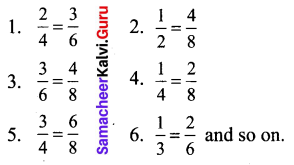# Samacheer Kalvi 6th Maths Solutions Term 1 Chapter 3 Ratio and Proportion Intext Questions

## Tamilnadu Samacheer Kalvi 6th Maths Solutions Term 1 Chapter 3 Ratio and Proportion Intext Questions

Recap (Textbook Page No. 55)Question 1.
Which of the following fractions is not a proper fraction?
(a) $$\frac{1}{3}$$
(b) $$\frac{2}{3}$$
(c) $$\frac{5}{10}$$
(d) $$\frac{10}{5}$$
Solution:
(d) $$\frac{10}{5}$$

Question 2.
The equivalent fraction of $$\frac{1}{7}$$ is ____
(a) $$\frac{2}{15}$$
(b) $$\frac{1}{49}$$
(c) $$\frac{7}{49}$$
(d) $$\frac{100}{7}$$
Solution:
(c) $$\frac{7}{49}$$

Question 3.
Write >, < or = in the box.
(i) $$\frac{5}{8}$$ ____ $$\frac{1}{10}$$
(ii) $$\frac{9}{12}$$ _____ $$\frac{3}{4}$$
Solution: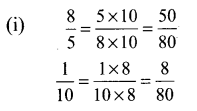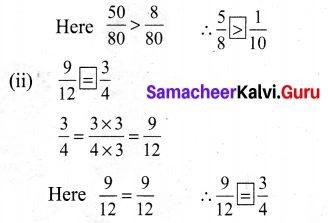Question 4.
Arrange these fractions from the least to the greatest: $$\frac{1}{2}, \frac{1}{4}, \frac{6}{8}, \frac{1}{8}$$
Solution:
$$\begin{array}{l}{\frac{1}{2}=\frac{1 \times 4}{2 \times 4}=\frac{4}{8}} \\ {\frac{1}{4}=\frac{1 \times 2}{4 \times 2}=\frac{2}{8}}\end{array}$$
Comparing $$\frac{4}{8}, \frac{2}{8}, \frac{6}{8} \text { and } \frac{1}{8}$$. we have $$\frac{1}{8}<\frac{2}{8}<\frac{4}{8}<\frac{6}{8}$$
i.e, $$\frac{1}{8}<\frac{1}{4}<\frac{1}{2}<\frac{6}{8}$$Question 5.
Annan says the $$\frac{2}{6}$$ th of the group of triangles given below are blue. Is he correct?Solution:
No, he is not correct because of the total of 6 triangles 4 are blue, i.e $$\frac{4}{6}^{\text {th }}$$ triangles are blue.

Question 6.
Joseph has a flower garden. Draw a picture which shows that $$\frac{2}{10}$$ th of the flowers are red and the rest of them are yellow.
Solution: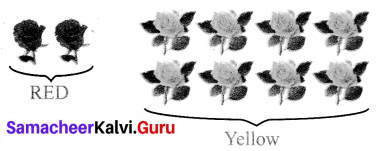Question 7.
Malarkodi has 10 oranges. If she ate 4 oranges, what fraction of oranges she was not eaten by her?
Solution:
$$\frac{\text { Oranges not eaten }}{\text { Total oranges }}=\frac{10-4}{10}=\frac{6}{10}$$

Question 8.
After sowing seeds on day one, Muthu observes the growth of two plants and records it. In 10 days, if the first plant grew $$\frac{1}{4}$$ th of an inch and the second plant grew $$\frac{3}{8}$$ th of an inch, then which plant grew more?
Solution:
Comparing $$\frac{1}{4}$$ th of an inch and $$\frac{3}{8}$$ th of an inch.
$$\frac{1}{4}=\frac{2}{8}<\frac{3}{8}$$
Second plant grew more.Try These (Textbook Page No. 57 to 60)

Question 1.
Write the ratio of red tiles to blue tiles and yellow tiles to red tiles.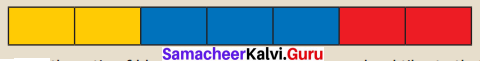Solution:
(i) Red tiles to blue tiles = 2 : 3
(ii) Yellow tiles to red tiles = 2 : 2

Question 2.
Write the ratio of blue tiles to that of red tiles and red tiles to that of total tiles.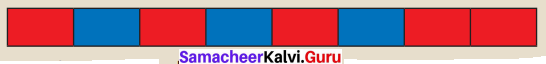Solution:
The ratio of blue tiles to red tiles = 3 : 5
The ratio of red tiles to total tiles = 5 : 8

Question 3.
Write the ratio of shaded portion to the unshaded portions in the following shapes.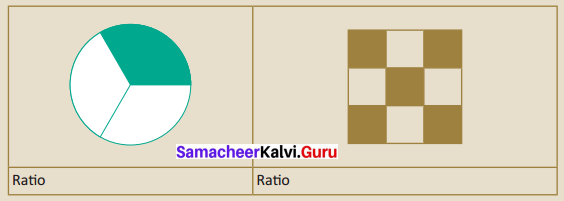Solution:
(i) Ratio = 1 : 2
(ii) Ratio = 5 : 4

Question 4.
If the given quantity is in the same unit, put ‘✓’ otherwise put ‘ ✗’ in the table below.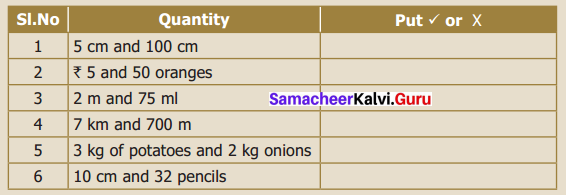Solution: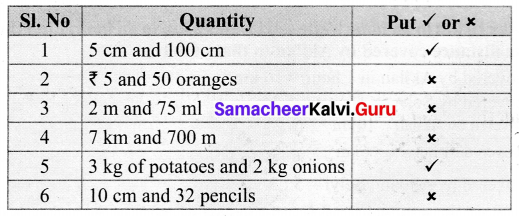Question 5.
Write the ratios in the simplest form and fill in the table.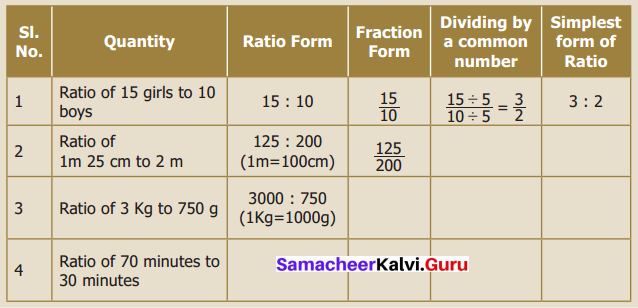Solution: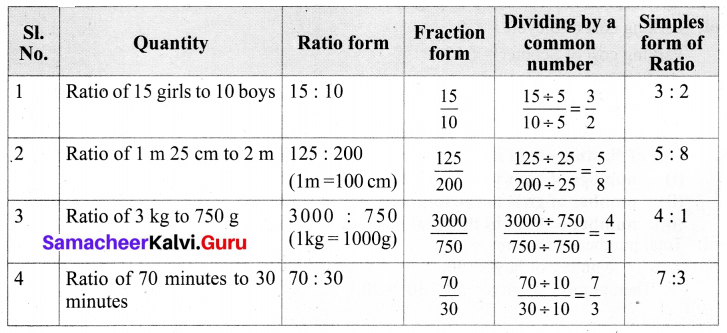Try These (Textbook Page No. 64)

Question 1.
For the given ratios, find two equivalent ratios and complete the table.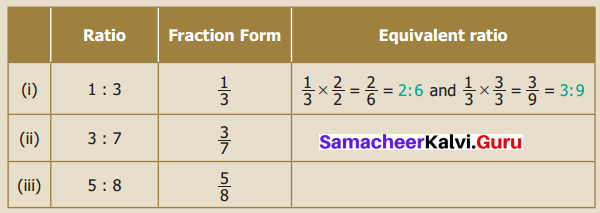Solution: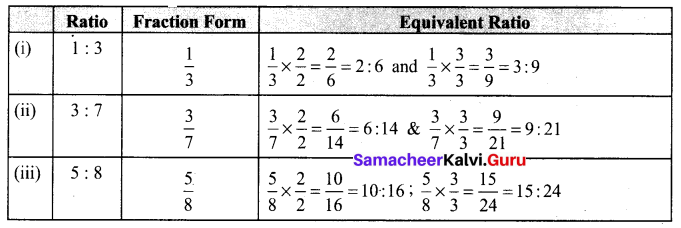Question 2.
Write three equivalent ratios and fill in the boxes.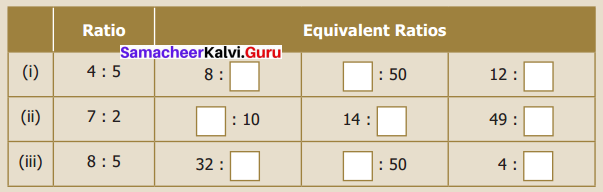Solution: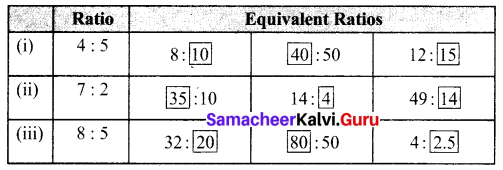Question 3.
Find the given ratios, find their simplest form and complete the table.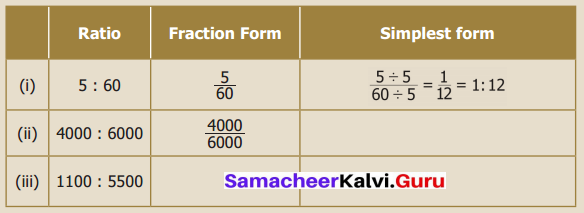Solution: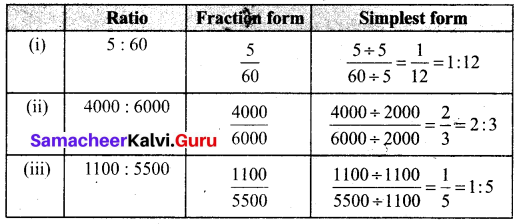Try These (Textbook Page No. 70)

Question 1.
Fill the box by using cross product rule of two ratios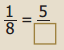Solution:By cross product rule we have 1 × □ = 5 × 8
1 × 40 = 40
∴ $$\frac{1}{8}=\frac{5}{40}$$

Question 2.
Use the digits 1 to 9 only once and write as many ratios that are in proportion as possible (For example $$\frac{2}{4}=\frac{3}{6}$$)
Solution: